Tool Switching Problem (ToSP)

ToSP involves scheduling a number of jobs on a single machine such that the resulting number of tool switches required is kept to a minimum.

This can be formalized as follows: let a ToSP instance be represented by a 4-tuple, I=(C, n, m, A) where,

·        C denotes the magazine capacity (i.e., number of available slots),

·        n is the number of jobs to be processed,

·        m is the total number of tools required to process all jobs (it is assumed that C < m; otherwise the problem is trivial).

·        A is a m x n Boolean matrix termed the incident matrix. This matrix defines the tool requirements to execute each job, i.e., Aij=TRUE if, and only if, tool i is required to execute job j.

The solution to such an instance is a sequence J1, …, Jn determining the order in which the jobs are executed, and a sequence T1, …, Tn of tool configurations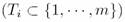determining which tools are loaded in the magazine at a certain time.

Processing each job requires a particular collection of tools loaded in the magazine. It is assumed that no job requires a number of tools higher than the magazine capacity, i.e.,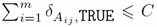for all j, where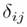is Kronecker's delta. The objective function F(.) counts the number of switches that have to be done for a particular job sequence

Datasets

As far as we know, no standard data instance exists for this problem (at least publicly available)  so that we have arbitrarily selected a wide set of problem instances that were attacked in (Bard, 1988)(Hertz,1998)(AlFawzan,2003)(Zhou,2005); more specifically, 16 instances were chosen with values for the number of jobs, number of tools, and machine capacity ranging in [10,50], [9,60] and [4,25] respectively. Table 1 shows the different problem instances chosen for the experimental evaluation where a specific instance with n jobs, m tools and machine capacity C is labeled as nm

Table 1. Problem Instances considered in the experimental evaluation. The minimum and maximum of tools required for all the jobs is indicated in second and third rows respectively. Fourth row display the bibliography reference from which the problem instance was obtained. (Bard, 1988)(Hertz,1998)(AlFawzan,2003)(Zhou,2005).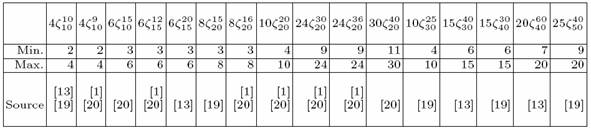Five different datasets (i.e., incident matrixes or relations among tools and jobs) were generated randomly per instance. Each dataset was generated with the restriction, already imposed in previous works such as (Hertz, 1998), that no job is covered by any other job in the sense that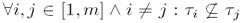where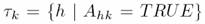is defined as before, i.e., the set of tools required to process job k. The reason to enforce this constraint is to avoid the simplification of the problem by preprocessing techniques as done for instance in (Bard, 1988) and (Zhou, 2005).

1. Format of datasets

Each file has follow structure.

The file is composing by n lines. Each line have follow information Ji:T1,T2,..,Tk#, where Ji  is a job, Tk  is a tool required by Ji  and # is a control character. For example,

0:1,6,0,4#

this indicate that job 0 required tools 1,6,0 and 4.

Additionally, the name file indicates the problem instances used, e.g., matrix_nj_mto_NSS_h, where h is a id of matrix.

Last update: 17 July 2008                                           Comments: jedgar@unet.edu.ve